# How to find all combinations of numbers that equal given sum in Excel

3 ways to find all combinations from a set of numbers that sum to a specific total.

Finding combinations of values that add up to a given sum is a common problem in data analysis. For example, you may want to know all possible combinations of items that can be purchased with a given budget, or all possible ways to allocate resources to meet certain requirements. In this article, we will explore how to use Excel Solver and VBA to accomplish the task. We will also discuss some potential pitfalls and limitations of each approach that may arise when handling large datasets or complex constraints.

## Find a combination of numbers equal to a given sum with Excel Solver

Regrettably, none of inbuilt Excel functions can help you identify the numbers that add up to a given total. Luckily, Excel provides a special add-in for solving linear programming problems. The Solver add-in is included with all versions of Excel but is not enabled by default. If you are not familiar with this tool, here's a great article on how to add and use Solver in Excel.

With the Solver add-in activated in your Excel, proceed with the following steps:

1. Create the model.

For this, enter your set of numbers in one column (A3:A12 in our case) and add a blank column to the right of your numbers for the results (B3:B12). In a separate cell (B13), enter a SUMPRODUCT formula similar to this:

`=SUMPRODUCT(A3:A12, B3:B12)`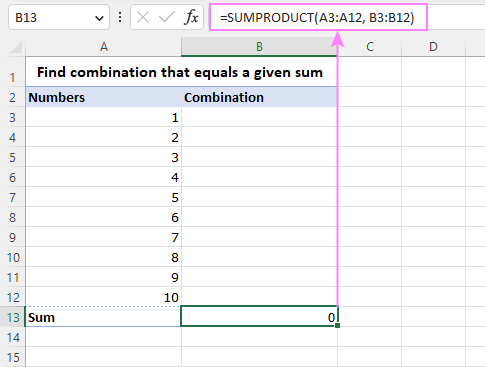On the Data tab, in the Analysis group, click the Solver button.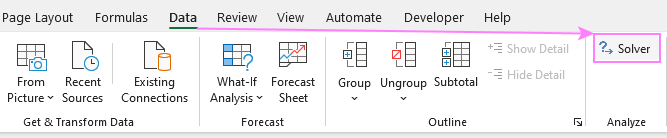3. Define the problem for Solver.

In the Solver Parameters dialog box, configure the objective and variable cells:

• In the Set Objective box, enter the address of the formula cell (B13).
• In the To section, select Value Of and type the desired sum value (50 in this example).
• In the By Changing Variable Cells box, select the range to be populated with the results (B3:B12).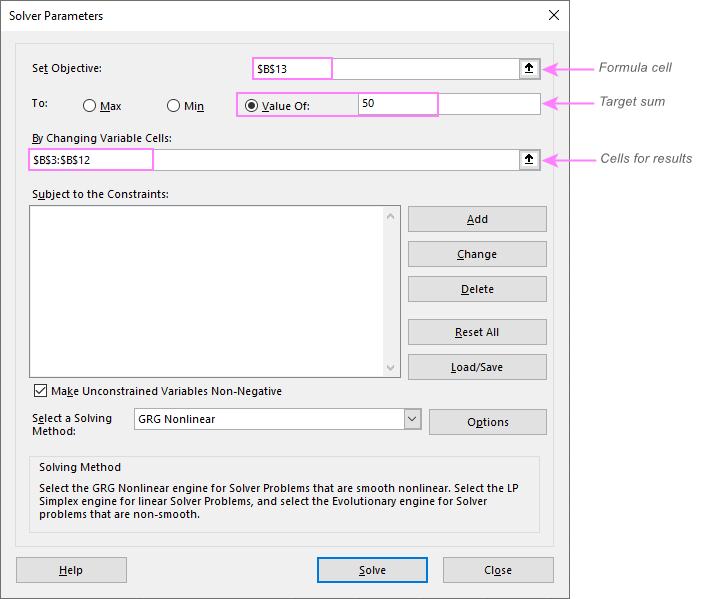To specify the constraints, i.e. the restrictions or conditions that must be met, click the Add button. In the Add Constraint dialog window, select the resulting range (B3:B12) and pick bin from the drop-down list. The Constraint will be automatically set to binary. When done, click OK.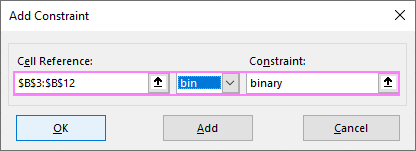5. Solve the problem.

When taken back to the Solver Parameter dialog window, review your settings and click the Solve button.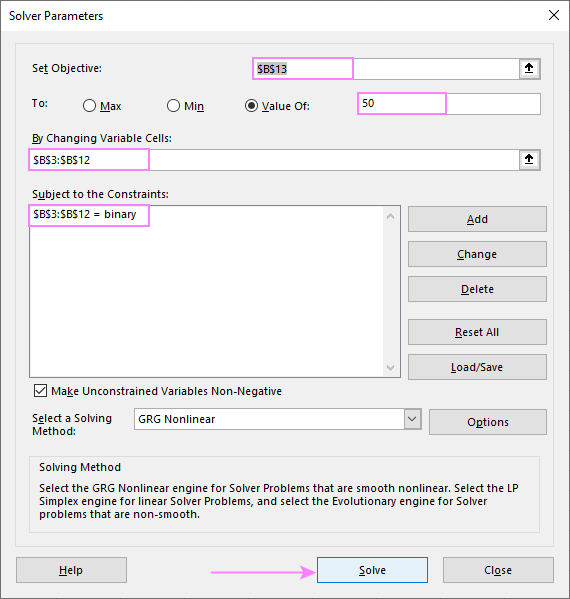A few seconds (or minutes) later, the Solver Results dialog box will appear. If successful, select the Keep Solver Solution option, and click OK to exit the dialog.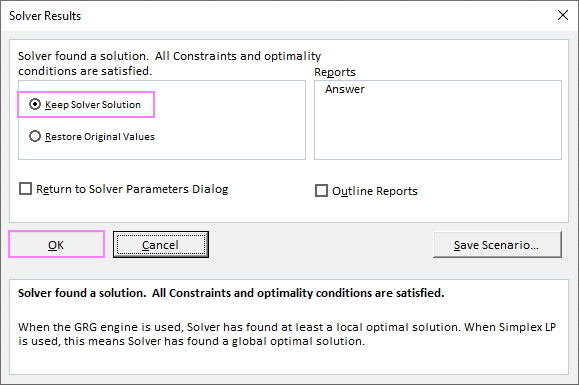As a result, you will have 1 inserted next to the numbers that add up to the specified sum. Not a user-friendly solution, but it's the best that out of the box Excel can do.

For visualization purposes, I've highlighted the cells that give the desired sum in light green: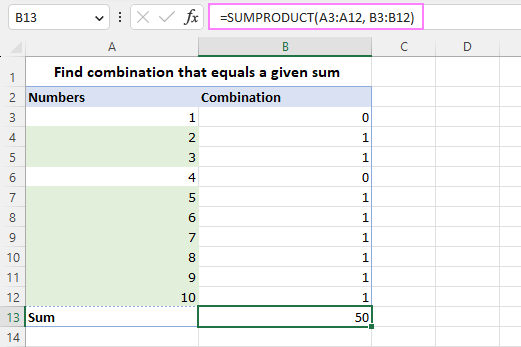Limitation: Excel Solver can find, at most, just one combination of numbers that equals a specific sum.

## Find all combinations that equal a given sum with custom function

To get all possible combinations from a given set of numbers that add up to a certain value, you can use the custom function below. If you are new to UDFs, you'll find a lot of useful information in this guide: How to create custom user defined functions in Excel.

How this function works

The main function, FindSumCombinations, calls a few subsidiary functions that implement smaller sub-tasks. The function named SumUpRecursiveCombinations executes the core algorithm that finds all possible sums in the specified range and filters those that reach the target. The ArrayToString function controls the form of output strings. Three more functions (SumArray, AddToArray, and CopyArray) are responsible for processing intermediate arrays: each time we create a temporary array, add one element from the source array to it, and check if the target sum is reached. The core algorithm is taken from this Stackoverflow thread, thank you guys for sharing!

Syntax

From the user's perspective, the syntax of our custom function is as simple as this:

FindSumCombinations(range, sum)

Where:

• Range is the range of numbers to test.
• Sum is the target sum.

Note! The custom function only works in Dynamic Array Excel 365 and 2021.

How to use the FindSumCombinations function:

1. Insert the above code into a Code module of your workbook and save it as a macro-enabled workbook (.xlsm). The step-by-step instructions are here.
2. In any blank cell, enter a FindSumCombinations formula and press the Enter key. Make sure there are enough empty cells to the right to output all combinations, otherwise the formula will return a #SPILL error.

For example, to find all possible combinations of numbers in the range A6:A15 that equal the sum in A3, the formula is:

`=FindSumCombinations(A6:A15, A3)`

As with any other dynamic array function, you enter the formula is just one cell (C6 in the image below) and it populates the results into as many cells as necessary. By default, the comma-separated strings are output in a row: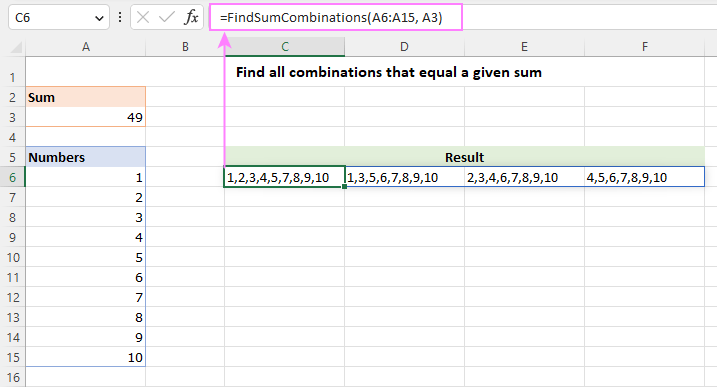To return the results in a column, wrap the custom function into TRANSPOSE like this:

`=TRANSPOSE(FindSumCombinations(A6:A15, A3))`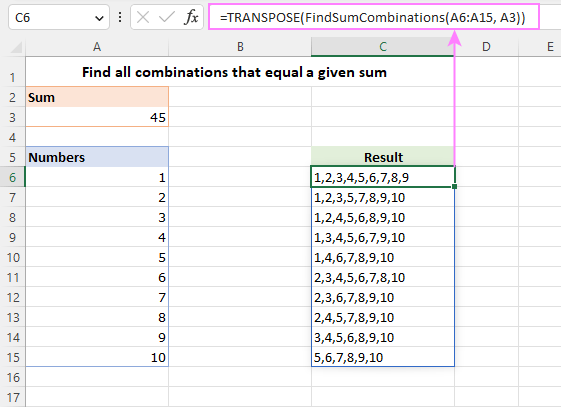To output the strings in the form of an array enclosed in curly braces, modify the ArrayToString function as follows:

The results will look similar to this: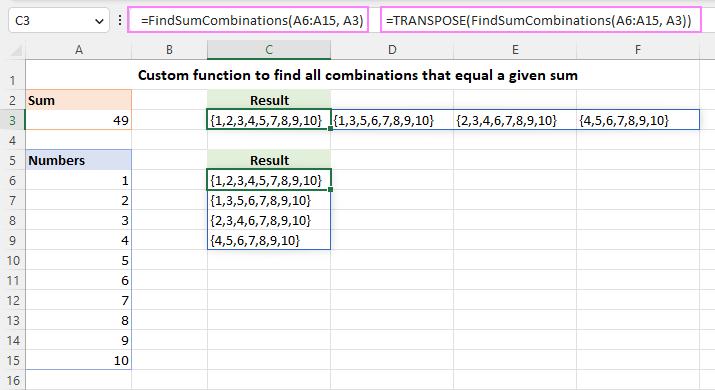Limitation: This custom function works only in Dynamic Array Excel 365 and Excel 2021.

## Get all combinations that equal a given sum with VBA macro

The custom function described above returns the combinations of numbers as strings. If you'd rather have each number in a separate cell, this macro will be helpful. The code is written by another Excel expert Alexander Trifuntov who has been actively helping users to solve various Excel problems on this blog.

Start by adding the following code to your workbook. For the detailed steps, please see How to insert VBA code in Excel.

Next, create a UserForm with the following design and properties: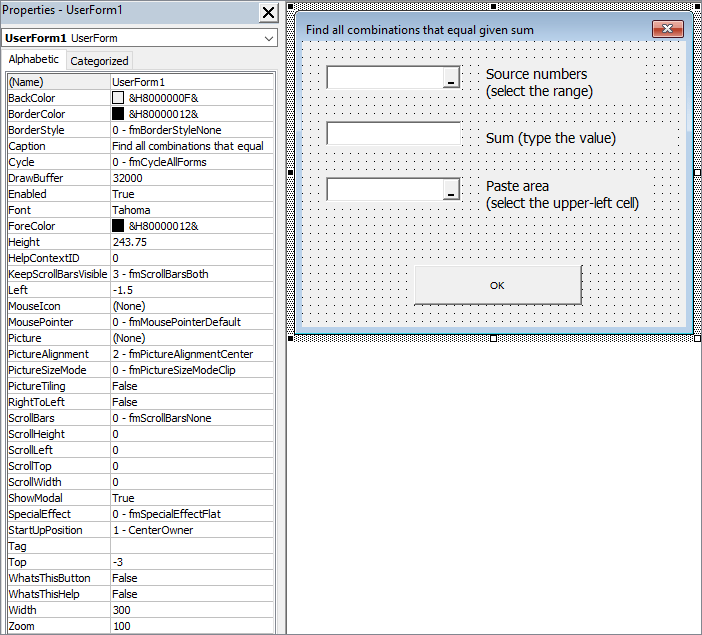After completing the form's design, add the code for the form. For this, right-click on the form in the Project Explorer and select View Code:

Tip. Instead of re-creating the form from scratch, you can download our sample workbook at the end of this post and copy the Code module and UserForm1 from there (see how to copy a macro to another workbook). To make the macro accessible in all your workbooks, copy it to the Personal Macro Workbook.

With the code and form in place, press Alt + F8 and run the FindAllCombinations macro: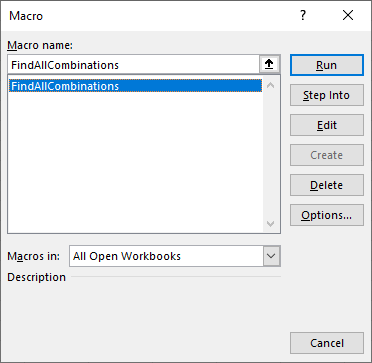On the form that pops up, define the following:

• The range with the source numbers (A4:A13)
• The target sum (50)
• The upper-left cell of the destination range (C4).

When specifying the upper-left cell of the output range, make sure there is at least one blank row above (for headers) and enough blank cells down and to the right. If there are insufficient blank cells, your existing data will be overwritten.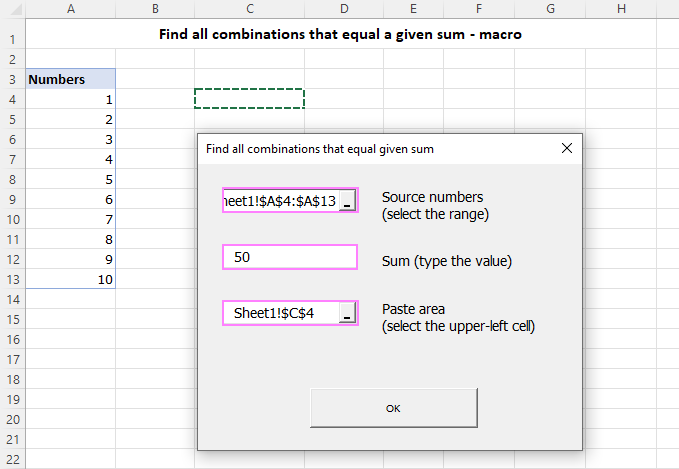Clicking OK will produce the result like shown in the screenshot below:

• In C4:C6, you have the combinations of numbers as comma-separated values.
• Columns F, G and H hold the same combinations of numbers, with each number residing in a separate cell.
• In D4, you have the target sum.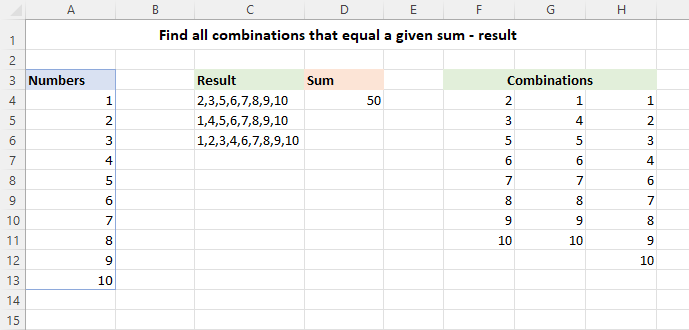This form of output makes it easier to check the result - just enter the SUM formula in cell F13, drag it to the right across two more cells and you will see that each combination of numbers adds up to the specified value (50).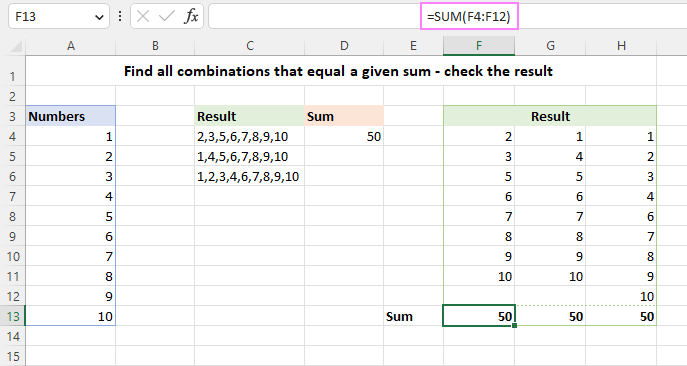Limitation: For a large set of numbers, the macro may take some time to generate all possible combinations.

Advantages: Works in all versions of Excel 2010 - 365; provides two forms of output - strings of comma separated values and numbers in separate cells.

In conclusion, finding all combinations of values that equal a certain value is a powerful tool for solving a wide range of data analysis problems. Hopefully, this article has provided you with a useful starting point for exploring this topic further and handling similar problems in your own work. Thank you for reading!

## You may also be interested in

1. I have 6 parameters (let’s call them A,B,C,D,E,&F) and each parameter can have a possible score of 1 thru 95. I am trying to determine all combinations of the 6 parameters that can sum to 100. How do I do this in EXCEL without EXCEL program crashing?

VR,

JPC

• Hi! Such a huge number of variants, Excel will not be able to calculate. This is 869107785 combinations of numbers.
You won't even be able to write them down on the worksheet.

2. I have no background experience with anything like this. I would love to get this macro to work properly. I get to a point of running the findsumcombinations macro, but keep getting "need object" error 424. Then when I go and re type in the formula my entire excel freezes up. Any help would be appreciated.

Thank you

• Hi! Regrettably, I was unable to replicate your issue, as I am unaware of the actions you take and the numbers you select. Please read carefully and follow the recommendations in the article above. Or describe the problem in detail.

3. In addition to my last comment, I am using and trying to find a negative number (I'm trying to see why there's a discrepancy between my internal math and my bank account). In a tiny experiment, it seems that negative numbers throw everything off, no matter how simple the list of numbers (I had a list which included -7 and -3, with a desired number of -10, and I still received a #VALUE! error). What should I do?

Thanks again!

4. Hello,

I followed all the steps correctly, to my knowledge, and received a "#VALUE!" error. The code I input into the cell was "=FindSumCombinations(A5:A194, A2)".

What should I check to troubleshoot this?

Thank you!

• Hi! A #VALUE! error means that no solution was found. Perhaps text values or empty cells were used. Do you really want to find all combinations of 189 numbers?

• Hi there, I also got the very same issue, but with strong possibility that some of the values are really close (less than 0,99 diff). but it only looks for exact matches as per example :
-FindSumCombinations(A1-A25,B2) where B2 might be by 0,99 bigger or smaller than B2 value.

I'll be more than thankful for everything there as I believe it will save me hundreds of hours of work during the year to come.

• Hi! I recommend using only integer numbers to find combinations, as Excel does not always work correctly with fractional part of a number. If you need to find a combination of numbers with two decimal digits, multiply them by 100 and then divide the numbers in the resulting combination by 100. If the numbers are the result of calculations, be sure to use rounding.

5. Hi, I've been struggling with this and can't seem to get solution. I'll appreciate your assistance.
I have a reference cell B1 to f1 with data 37, 65, 20, 8 and 24 in the cells respectively.
How do I make excel do a two cell combination of all the 5 cells. Eg. B1+E1 which is 37+20 and then compare with the rest of the worksheet to find all two cells on a row with equal sum total of any of the combinations above. If found, set same border color for cells with equal sum total.
I tried pairing but it only found cells that were close together. Eg. Cells B22 and C22 with sum total of 28.
If possible, I can share my worksheet with you via email so you can see what I mean.

• Hi! You need to write a special VBA macro for your task. This goes beyond the advice we provide on this blog.

• I have written the vba macro but it's not working as intended.
Thanks for your time anyway 🙂

6. This was TREMENDOUSLY helpful and I learned something new today about the Solver add in. Thank you

7. Hi,
I've tried the solutions and don't seem to figure out how to make them work. With macro I get debugging popping up.
I have a number of incoming invoices and data from bank, that has only total sums of those invoices and how many of them were matched to the sum. So I need to figure out what is what, seems that Solver should do the trick.. But it's running literally forever. I've added limitation based on the amount of invoices, didn't help. The amount of initial variables is below 70, it should be ok then..
I had to restart the pc everytime to exit excel because it's not responsive and just running the solver, doesn't seem right at all :D

• Unfortunately, this information is not enough to understand what you need.

8. How do I sort the list in excel to 0.01 miles - 10 miles, 10.01 miles - 20 miles, 20.01 miles - 30 miles, 100.01 miles - 110 miles, 120.01 miles - 130 miles, 110.01 miles - 120 miles instead of 0.01 miles - 10 miles, 10.01 miles - 20 miles,100.01 miles - 110 miles, 120.01 miles - 130 miles, 110.01 miles - 120 miles, 20.01 miles - 30 miles

Thank you

9. I want a random combination of my sum but not all the combination one is enough. But I have ample data, so when I use your function my Excel freezes because it looks for all the combinations. How do I stop that?

10. I wonder if we could allow repetition of numbers in the combination in order to make possible a better approach of the final sum? How would that be possible? For exemple, if we consider a set of numbers 1,2,3,4 and we want to look for combinations that sum up to 20 we must allow he numbers in the combination to be repeatable is this case included in this approach by SOLVE function?

• Hi! I think you can make repeats in your set of numbers. Then those repeats can be in the final result.

11. Hi, my computer can't handle the large arrays I want to calculate. I also do not need combinations of >4-5 values to sum my desired value. Is there a way to edit this function to only search for a maximum set of values to sum to 1) match my needs better 2) to lessen the burden on my computer?

Ex:
(array: 1,2,3,4,5,6,7,8,9,10,11,12,13,14,15) (sum target: 24)
desired yield: (15,9), (6,7,9), etc
undesired/wasteful yields: (1,2,3,7,9)

• Hi! In order to select the part of the values you want, Excel must calculate all values. Therefore, your request is not possible.

12. Hi, am trying to use the macro to work with dollar amounts (dollars and cents) and am getting a runtime error. Using whole integers works fine if the data entered does is not greater than .00, all other amounts will compile an error. Is there a way to alter the code to include decimals. For instance, search for \$1043.02 from the following data.

\$20.67
\$129.61
\$193.26
\$74.34
\$66.61
\$188.67
\$125.96
\$132.79
\$169.81
\$99.73
\$95.80
\$339.79
\$410.21
\$293.02
\$142.90
\$298.89
\$262.48
\$1,611.81
\$384.78

• Hello! This question has been asked before, if you've read the comments. The function works with integers. You can multiply all your numbers by 100.

• Yes, indeed I did see that, but I was asking how to alter the code to avoid the workaround itself. When altering the search values and the target value by 100 factor, a run-time error 6 "overflow" results with the debug line pointing to "TargetSum = TextBox1.Value" The search seems to work find for smaller integers, but for the larger numbers error out. Otherwise this is great, because it seems to also take negative values into consideration, which is perfect for the scenario I am trying to use if the larger numbers work.

• To further explain, if you look at the first 5 values I provided and alter them to

2067
12961
19326
7434
6661

Their sum is 48449. When using this value in the target value box, the mentioned runtime error appears.

• Hello! We changed the macro code to work with larger numbers. Install the code again.

• Thanks for looking into this. I can't seem to get it to work while changing the code, perhaps I am not editing everything correctly. Could you upload a new sample workbook to work off of with the changes you've made? I appreciate your time!

• Hi! New sample workbook uploaded.

• Thank you! It seems to run in the background now but is freezing up my excel every time. I've tried with the previous example I gave earlier, as well as a sum of just 2 numbers to see if it was just a calculation/processing issue on my end with no luck - just crashes. I'm assuming the sample workbook works fine for you?

• Hi! The speed of Excel depends on such things as the formatting of your data, other processes running at the moment, available system resources, RAM, etc. We don't have any problems with this file.

• I assume general, currency, and number formatting for cells should work the same or is there one cell format we should be sticking to?

• Hi! Unfortunately, I didn't understand your question. You can use any custom data format you want.

13. Hi! Thank you so much for the great work. Whenever I try to run the macro function (copied from the example file) I receive a "Run-time error 6: Overflow" message. Thanks again!

• Hi! Unfortunately, I can't see what you're doing or what data you're using. Try placing your data in a sample file and find the combination of numbers.

14. Can you please change the code so the output are only groups of 5 integers which satisfy the same requirement?

• We give you all the results, so you can choose the right ones for you.

15. Hello, I am using the findsumcombinations function and it is rounding to the nearest dollar, when I need it to be exact on the penny.

Thoughts on this?

thank you!

• Hello! The function works with integers. You can multiply all your numbers by 100.

16. hello,

we have steel coil slitting equipment. we are required to have minimum wastage from our slitting plan. lets keep that at 0-5mm from the coil width. suppose the coil width (which differs) here is 1219mm and considering the minimum wastage we have 1214mm remaining from which we can take as many slits as required from a list of slit sizes.
e.g 121mm / 240mm / 35.70mm / 115mm / 95.4mm (with decimals)

now i need a best combination list, multiples of each size allowed, where the total of the selected size should not exceed 1219-5=1214mm

kindly advice how can this be achieved.

• Hi! Since your numbers can't give an exact sum of 1214, use Solver. You will be able to find the best combination of numbers that is close to 1214.

17. Hello,

I did a simple Bing search for "Find all combinations of a number..." and it led me to this post. Excellent work, I love the simplicity and easy instructions. I will dabble with the UDF in a bit, but for now, could you tell me how to do the above method and have the following additional information.

Not only show a 1, but also append the times it needs to be multiplied after adding the other numbers in the array.

For example

Desired total would be 50
Left array is 1 through 10
The empty column results would show 1 x10 next to the 5
The 1 indicates true, while the x(number) indicates multiples needed

Of course, the 10 would be 0 in the right array because 5x10 already reaches the desired total.

Please let me know if this makes sense.

• Hi! This custom function and macro do not assume that the numbers will be used more than once. Also, they show all possible combinations of numbers. For your case, it is better to use Solver.

18. Hi there,
First I would like to thank you very much for this great work,
I was looking for this solution for a while since I work in accountancy and I struggle to find the possible solutions or combinations that add up to a given value say payment.
I have one question to ask if you don't mind,
in the VBA macro, I don't see anything to change like a range or a sheet name,
My question is, does it work on any workbook as it is ?
in other words, would it work if I copied it to my workbook with my tables and values or is there anything to modify ?
Great job.
May God bless you.

• Hi! Copy the code into your workbook as described in the article above. Remember to save it as an .xlsm
The macro will work with your data.

19. By the way great job. May God bless you.

20. Hi. I am using findsumcombinations formula, even there should be more than 1 result, excel gives me only one of them. Is there any solution for that?

• Hi! Unfortunately, this information is not enough to give you any advice. What source data and what formula did you use?

• Just I wrote 1, 2, 3, 4, 5 in each sells and my formula is "=FindSumCombinations". The sum should be 10. The only option excel gives is 1, 2, 3, 4. While we have 1+4+5 for example. It gives only one result.

• 10 is in cell D10. The numbers 1,2,3,4,5 are in cell D12, D13, D14, D15, D16. And my formula is : =FindSumCombinations(D12:D16;D10)

And the result is only "1,2,3,4"

• Look carefully at your formula. I'm sure you only used numbers 1,2,3,4. The formula FindSumCombinations with numbers 1,2,3,4,5 gives 3 results {1,2,3,4} {1,4,5} {2,3,5}

21. Hi Newbie here, May I know why after I press Alt + F8 and run the FindAllCombinations macro it show Run-time error '424' and ask for Debug. Did I skip a step

• Hi! I cannot see all of your actions. You may not have followed all of the instructions in the article above. Download the example file from the link at the end of the article.

22. I am struggling with nimber with decimals. Like money amounts. How can I get it to work with decimals.

• Hi! Your task is not completely clear to me. Maybe this article will be helpful: Custom Excel number format. If this does not help, explain the problem in detail.

• When I am looking for an amount with a decimal point as 788.39
And the numbers I'm working with is 45.35, 54.98, 526.35, 54.34, 98.36, 54.36, 59.47, 85.64, 98.63, 78.36 It gives me an error.

But if i use 45.00, 54.00, 526.00, 54.00, 98.00, 54.00, 59.00, 85.00, 98.00, 78.00 Looking for 788.00 it will work.

What do I need to format so when the number does have a decimal point it will work.

Thank you very much for your help.

• Hi! I can't guess which error you are getting. Also note what decimal separator Windows uses. Also check if some number is written as text.

• It is designed for integers coz they are using Long data type

23. Thank you for putting together this great tutorial and example. Out of the many out there, yours is most effective while also being easy to follow. Thank you! Also, I must say that you have the patience of saints to continue answering nearly the same exact questions from people who don't follow the tutorial and therefore cannot apply it to their specific situation. Thanks again for sharing your brilliance with the world!

24. I need select 6 unique numbers from a range say (1 to 45) that the sum is as specified say 165
What is the formulae for all possible combinations (I know it is 72,974, but I don't know how to get it)?
How to generate all the possible 6 number combination.
Regards
Steven

• Hi! You can use the function or macro from the article above, if your Excel has the technical capabilities to perform these calculations.

• Alexander,
Thanks for your response, but the spread sheet uses all numbers required to get the sum.
I need to specify the number of selected numbers say 6, from a pool of 45 to give the required sum, say 165
Any Idea on the formula, for all possible combination of say 6 unique numbers from a specified range say 1 to 45, to give the specified sum, say 165?

Regards, Steven

• Hi! Unfortunately, these features are not available in the proposed macros.

25. Hi, may I ask if column A is combines data separate with comma and the value is 1,2,3 and column B is another cell with a combined date 7,8,9 . How can I come up with the formula to get the results of the first number show in column A to multiply the first number in column B and so on for example like (1*7)+(2*8)+(3*9)? Thanks.

26. For a large data sets, Excel goes "Not responding" and I have observed that it uses only 2 thread of my 12 thread CPU, how can we tweak it to support multi threading.

• Hi!

• Hi Alexander,

Thank you for your prompt response, even though it is enabled, excel still uses 2 threads.
Also, how can we make changes to the formula if we wish to find the combinations using a set of specified number. For example, if we have 14, 80, 6, 60, 15, 25, 90, 3, 7 and we want to find all sets of 3 which would add upto 100

• Use the tools presented in this article to find all combinations of numbers and select the ones you want.

• I somehow managed to solve the set requirement. Would greatly appreciate if you could help with multi-threading challenge

27. Suppose I have a list of range as follows:

15
25
32
53
85
45
14
46
45
32
40
85

I wish to get the combination of all possible numbers which on adding gives me the value between 145 to 150 (inclusive both).

There can be multiple combinations possible which gives me the value between 145 to 150 but find all of them manually is not so easy.

Is there any way we can get my required result through Excel .

• Hi!
Find all possible combinations for each of the numbers in the range you specify with a custom function or macro.

• Could you send me that micro code.

• This VBA code is in the article above.

28. How to find all combinations of numbers that equals (say, 25000) or falls between a range (say, 22,500-25,000) of given sum in Excel.

Is it possible to find the sum of numbers that is within a range ?

If yes please tell me how.

• To find all combinations of numbers that equal a given value, say 25000, use one of the methods described in this tutorial. For finding a sum that falls within a range, we don’t have a ready-made solution at the moment, sorry. Writing such code will take a while.
To find the sum of numbers within a range, use the SUM function :)

• Hi!
Have you tried the ways described in this blog post? Please re-check the article above since it covers your case.